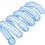# Answer to a Question Recently Posed

Someone asked this question recently:

"Two particles are simultaneously performing SHM along the same path with same time period and equal amplitude A. If the maximum separation b/w particles if (root3)A, then find their phase difference and positions when they cross each other."

This question involves some interesting mathematics. Consider the complex representation of a sinusoid ("Re" denotes "real part" and "j" is the imaginary unit):

$A \, cos(\omega t) = Re[A e^{j \omega t}]$

Notice that the coefficient on the exponential term is the magnitude of the sinusoid. That will be important to remember. Write general expressions for the two sinusoids under consideration:

$f_1 = A \, cos(\omega t) \\ f_2 = A \, cos(\omega t + \theta)$

Take the difference:

$f_1 - f_2 = A \, cos(\omega t) - A \, cos(\omega t + \theta) \\ = Re[A e^{j \omega t}] - Re[A e^{j (\omega t + \theta)}] \\ = Re[A e^{j \omega t} - A e^{j (\omega t + \theta)} ] \\ = Re[e^{j \omega t} (A - A e^{j \theta}) ]$

We can therefore infer that the magnitude (peak value) of the resultant sine wave is the magnitude of the complex quantity $A - A e^{j \theta}$, which we also know to be $\sqrt{3} A$. The peak value of the resultant sine wave will occur when the complex exponential has a phase angle that is the negation of the phase angle of $A - A e^{j \theta}$, making the argument of the Re() operation simply equal to the length of $A - A e^{j \theta}$. The following therefore must be true:

$|1 - e^{j \theta}|^2 = 3 \\ (1- cos\theta)^2 + (sin\theta)^2 = 3 \\ -2 cos\theta = 1 \\ \implies cos\theta = -\frac{1}{2}$

The two sinusoids are therefore separated by plus or minus 120 degrees. The other timing information should be fairly easy to figure out from here.Note by Steven Chase
3 years, 7 months ago

This discussion board is a place to discuss our Daily Challenges and the math and science related to those challenges. Explanations are more than just a solution — they should explain the steps and thinking strategies that you used to obtain the solution. Comments should further the discussion of math and science.

When posting on Brilliant:

• Use the emojis to react to an explanation, whether you're congratulating a job well done , or just really confused .
• Ask specific questions about the challenge or the steps in somebody's explanation. Well-posed questions can add a lot to the discussion, but posting "I don't understand!" doesn't help anyone.
• Try to contribute something new to the discussion, whether it is an extension, generalization or other idea related to the challenge.
• Stay on topic — we're all here to learn more about math and science, not to hear about your favorite get-rich-quick scheme or current world events.

MarkdownAppears as
*italics* or _italics_ italics
**bold** or __bold__ bold
- bulleted- list
• bulleted
• list
1. numbered2. list
1. numbered
2. list
Note: you must add a full line of space before and after lists for them to show up correctly
paragraph 1paragraph 2

paragraph 1

paragraph 2

[example link](https://brilliant.org)example link
> This is a quote
This is a quote
    # I indented these lines
# 4 spaces, and now they show
# up as a code block.

print "hello world"
# I indented these lines
# 4 spaces, and now they show
# up as a code block.

print "hello world"
MathAppears as
Remember to wrap math in $$ ... $$ or $ ... $ to ensure proper formatting.
2 \times 3 $2 \times 3$
2^{34} $2^{34}$
a_{i-1} $a_{i-1}$
\frac{2}{3} $\frac{2}{3}$
\sqrt{2} $\sqrt{2}$
\sum_{i=1}^3 $\sum_{i=1}^3$
\sin \theta $\sin \theta$
\boxed{123} $\boxed{123}$

## Comments

There are no comments in this discussion.

×

Problem Loading...

Note Loading...

Set Loading...